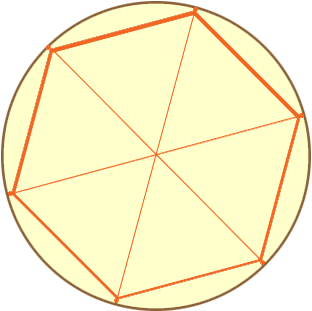Subject: Creating a triangle in a circle Name: Dirk Who are you: Parent My daughter has a school project where she must draw a circle and then draw an equilateral triangle inside the circle. She said you have to identify six points on the circle to correctly draw the triangle. How do you accomplish this? Hi Dirk, Suppose that you have a regular hexagon and you take a compass, put the point at the centre of the circle, put the pencil point at one of the vertices of the hexagon and draw a circle. Join the centre of the circle to each vertex.Each of the triangles in the diagram is an equilateral triangle and hence the sides of the hexagon have length equal to the radius of the circle. Now lets make the construction of a hexagon inside a circle by drawing the circle first. Draw a circle. With the compass width still the radius of the circle put the point at some point on the circumference of the circle. Draw an arc that intersects the circle. Move the compass point to the point where the arc intersects the circle and draw another arc that intersects the circle. Move the compass point to this point and repeat. After six repeats you are back to the point on the circle where you started. (This is quite difficult to do accurately and you may not end exactly where you started.) With a ruler connect, in order, the points you found on the circle. Now you have constructed a hexagon inside the circle. Can you see how to use this to get a triangle? Penny